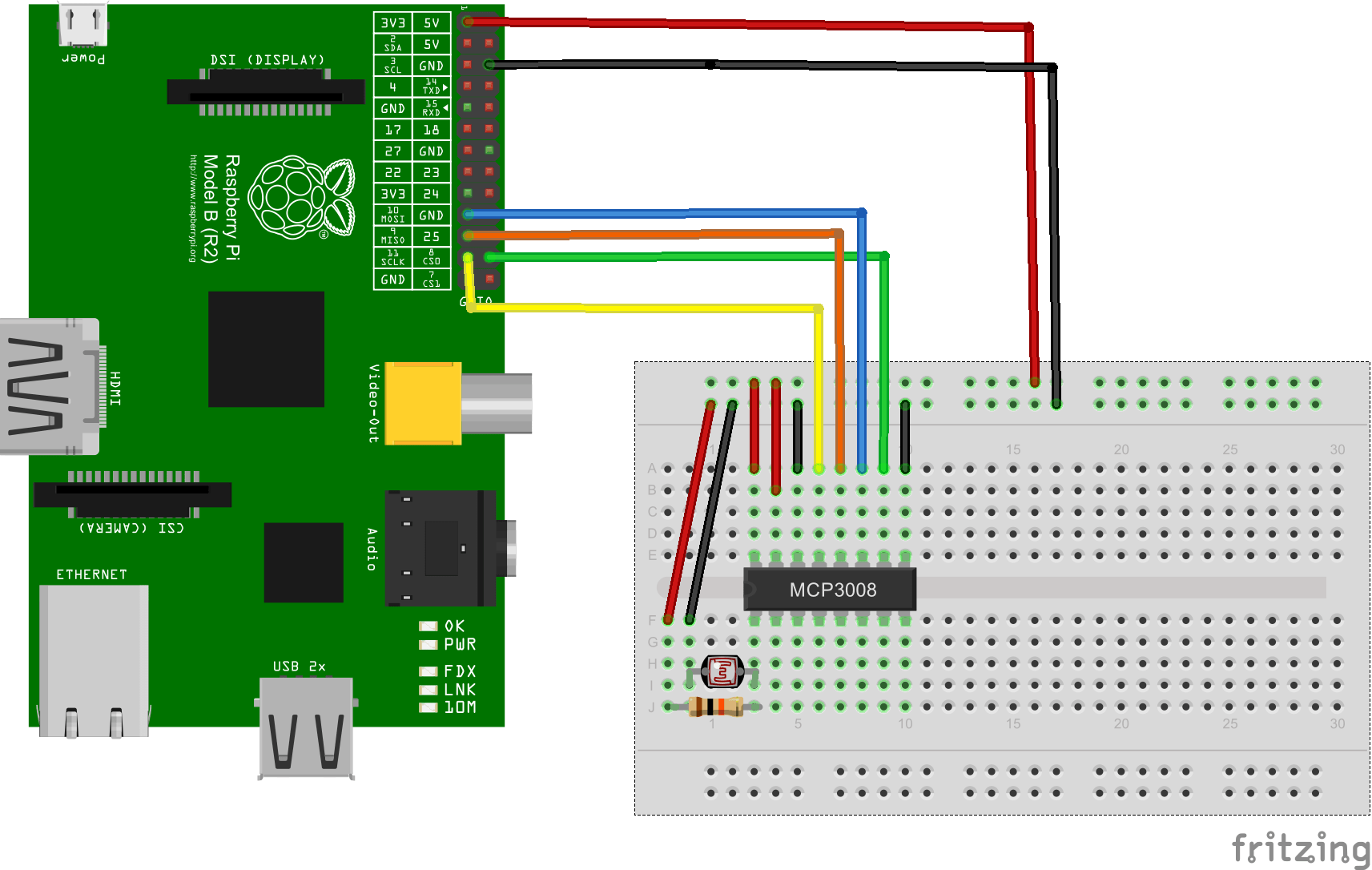Home Code Analogue inputs on the Raspberry Pi using an MCP3008

# Analogue inputs on the Raspberry Pi using an MCP3008

One of the frustrating things about the Raspberry PI is the lack of any analogue inputs, this means there are a number of sensors that are commonly used that cannot be used quite so easily on a Raspberry PI. Examples of these are light dependent resistors, LM35 and TMP36 temperature sensors.

Luckily it is quite easy to add this functionality by connecting a Analogue-to-digital converter (ADC) to you Raspberry PI. On this page we will connect a MCP3008 10bit 8-channel Analogue-to-digital converter. The MCP3008 connects to the Raspberry PI using the SPI bus (you’ll see this in the breadboard layout)

Lets look at the pinout of the MCP3008

You connect it like this

Layout

Here is the layout, connect the LDR to CH0 (Pin 1). I used a breakout.Code

Copy this as temp.py in an editor, I like to use Geany because you can execute your python scripts as well

```Source codeimport spidev
import time
import os

spi = spidev.SpiDev()
spi.open(0,0)

data = ((adc&3) << 8) + adc
return data

def ConvertVolts(data,place):
volts = (data * 3.3) / float(1023)
volts = round(volts,place)
return volts

ldr = 0

delay = 5

while True:
ldr_volts = ConvertVolts(ldr_value,2)

print "--------------------------------------"
print("Light : {} ({}V)".format(ldr_value,ldr_volts))

time.sleep(delay)```

Results

Run the example above and you should see something like the following. Cover the ldr and shine a bright light on it to change the reading

Light Dependent Resistor LDR 5MM Photoresistor